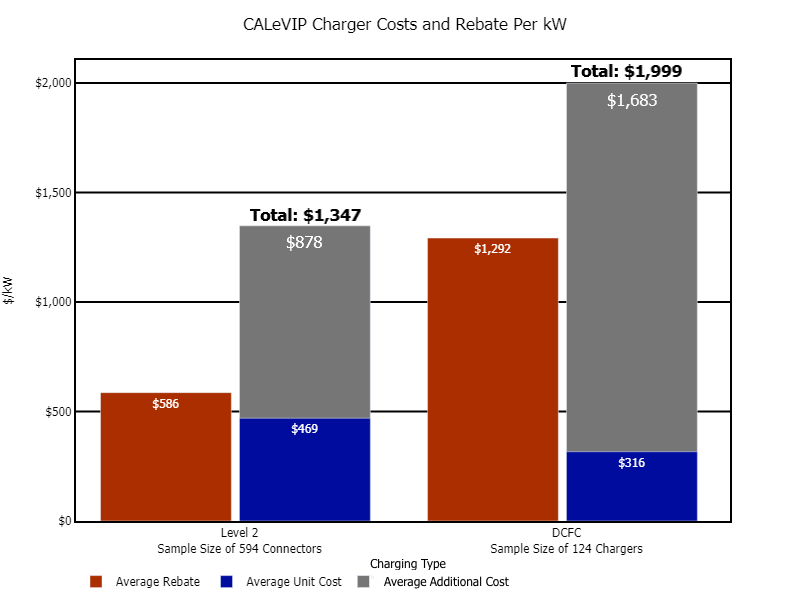The bar graph shows the average rebate paid per rated kW, the average unit cost per rated kW, and the average total project cost per rated kW installed by charging type: Level 2 and Direct Current (DC) fast charger.

For Level 2 chargers, the average rebate per rated kW was \$586, the average total project cost per rated kW was \$1,347, and the average unit cost per rated kW was \$469. For DC Fast Chargers, the average rebate per rated kW was \$1,292, the average total project cost per rated kW was \$1,999, and the average unit cost per rated kW was \$316 This graph excludes sites where the site host installed both Level 2 and DC Fast Chargers under a single rebate. This graph excludes some DC fast charger sites where the unit cost was undefined because of aggregated equipment costs.